# Bayesian Decision Science¶

Adapted from Ravin Kumar's PyData Global 2020 talk on Bayesian Decision Science (Youtube)(Github), updated with pymc v4.0 and some notes on learning the new syntax.

His original talk was done with pymc3, and since then pymc3 was rebranded as pymc and then they hit version 4.0 recently, which introduced some big changes.

In :
import matplotlib.pyplot as plt
from scipy import stats, optimize
import numpy as np
import pandas as pd
# np.set_printoptions(precision=2, floatmode="fixed")
import arviz as az

%config InlineBackend.figure_format='retina'


Note the new way of importing pymc:

In :
import pymc as pm

print(f"Running on PyMC v{pm.__version__}")

Running on PyMC v4.0.0


## Newsvendor problem with fixed demand¶

Next few cells follows Ravin's example of a newsvendor problem. First section he discusses a very simple example where demand is fixed, then you can simply write out the problem in code below:

In :
newspaper_cost = 5
customer_price = 7

anishas_inventory = 42
demand = 40

profit = demand*customer_price - newspaper_cost*anishas_inventory

f"Anisha makes ${profit} in profit"  Out: 'Anisha makes$70 in profit'

The next point is that you can use numpy and turn this into a function:

In :
def daily_profit(inventory, demand, newsvendor_cost=5, customer_price=7):
"""Calculates profit for a given day given inventory and demand"""
return customer_price*np.min([inventory, demand]) - newsvendor_cost*inventory

In :
f"Decision 1: profit ${daily_profit(42, 40)}, Decision 2 profit${daily_profit(38, 40)}"

Out:
'Decision 1: profit $70, Decision 2 profit$76'

### Optimization with scipy.optimize¶

So he then demonstrates that you could just plot a whole bunch of values of inventory and graphically inspect where profit is maximized, but we can do this by turning it into an optimization problem using scipy.optimize.

In :
# Restating here so future slides won't have the print
def objective(inventory: int, demands: iter):
"""Takes an iterable of sales and returns the total profit"""
# Make reward function negative to turn this into a minimization problem
return -np.sum([daily_profit(inventory, d) for d in demands])

In :
# Let scipy find minimie objective function (negative profit) if demands is set to 40
opt_stoch = optimize.minimize_scalar(objective, bounds=(0, np.inf), args=([40, 40]))
opt_stoch

Out:
     fun: -159.9999981773509
message: '\nOptimization terminated successfully;\nThe returned value satisfies the termination criteria\n(using xtol = 1.48e-08 )'
nfev: 43
nit: 38
success: True
x: 40.00000018226491

Great, so we have an optimal objective value of -160 (i.e. \$160 in profit) and it found the optimal inventory is 40. Makes sense - if demand is exactly 40 then you optimize profit when you buy and sell exactly that number of newspapers. ## What if demand is uncertain¶ But real life is a little more complicated, so what if we simulate random demand? Here, we simulate that demand is drawn from a normal distribution:$\mathcal{N}(40,20)\$

In :
np.random.seed(seed=0)
random_seeds = [1,2,3,4,5]
demand = np.round(stats.norm(40, 20).rvs(15))
demand_seen, demand_unseen = demand[:5], demand[5:]

In :
f"The first five days demand we have seen {demand_seen}"

Out:
'The first five days demand we have seen [75. 48. 60. 85. 77.]'
In :
f"The UNSEEN next ten days of demand {demand_unseen}"

Out:
'The UNSEEN next ten days of demand [20. 59. 37. 38. 48. 43. 69. 55. 42. 49.]'

## Instability in the sample mean and std deviation¶

He then goes on to use examples of taking the sample mean (the mean of the 5 days of demand that was seen) and the critical fractile equation to calculate the optimal inventory strategy.

I included his next point here, which is that 5 samples (i.e. seeing only 5 days of demand) can have wide differences in sample mean and std deviation. Very nice plot.

In :
simulations = 100
mean, std = [], []

for _ in range(simulations):
true_mean,true_std = 40, 20
values = stats.norm(40, 20).rvs(5)
mean.append(values.mean())
std.append(values.std())

In :
fig, ax = plt.subplots(2,1, figsize = (18, 8))

ax.plot(range(simulations), mean)
ax.set_xticks([])
ax.axhline(40, 0, 1, c="black", linestyle="--")
ax.set_ylabel("Estimate of Mean")

ax.plot(range(simulations), std)
ax.axhline(20, 0, 1, c="black", linestyle="--")
ax.set_xlabel("Calculation iteration")
ax.set_ylabel("Estimate of Std")

ax.set_title("Instability in Sample Mean and Standard Deviation from just 5 samples");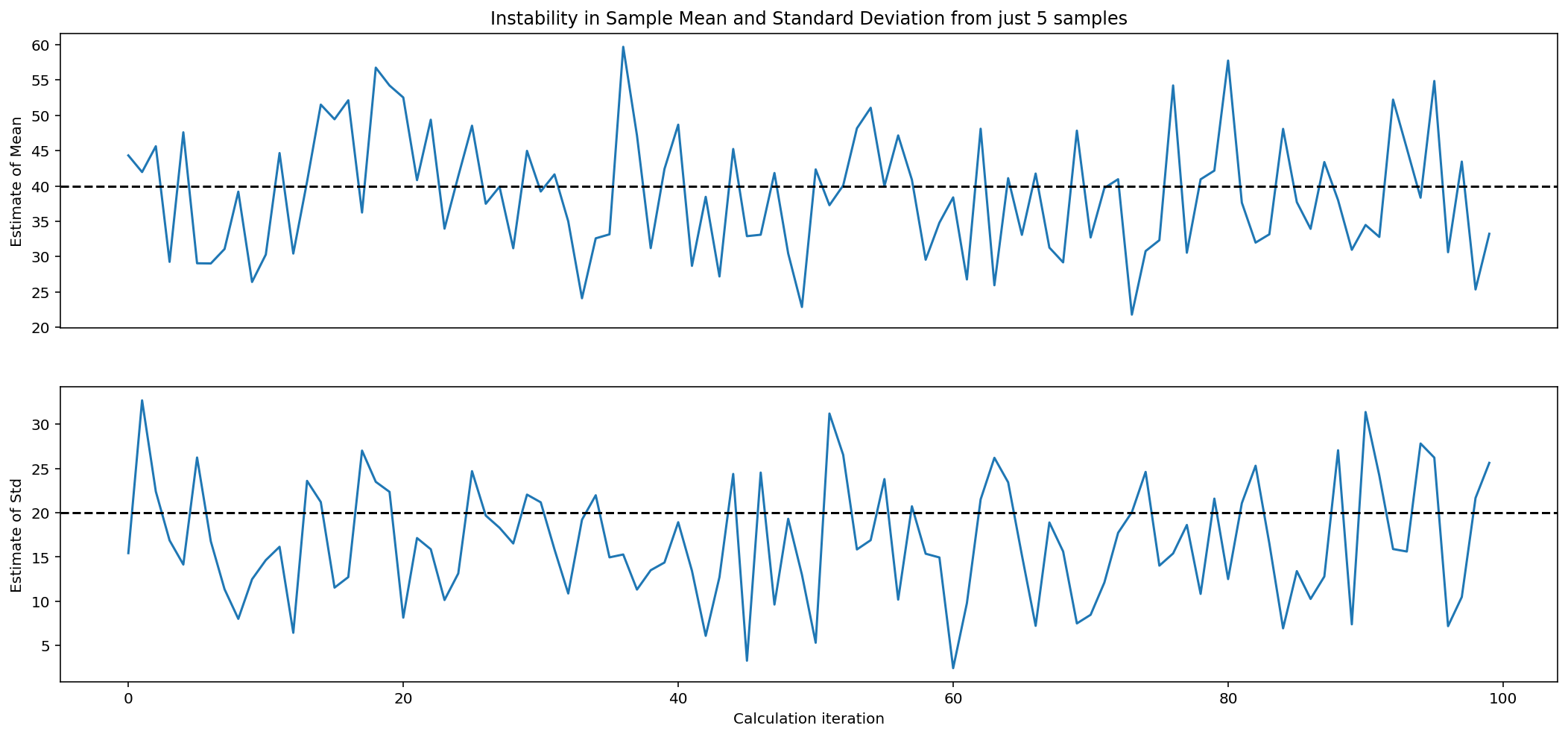## Enter Bayesian Modeling¶

Bayesian Modeling offers advantages because it handles this low sample size problem natively. He doesn't discuss how to choose informative priors and all that, but in this case he goes ahead and specifies the generative model below:

\begin{aligned} \sigma &\sim \text{HalfStudentT}(\sigma=10, \nu=20) & \text{Prior} \\ \mu &\sim \mathcal{N}(\mu=\bar{x}, \sigma=20) & \text{Prior} \\ \text{Demand} &\sim \text{TruncatedNormal}(\mu, \sigma) & \text{Likelihood} \end{aligned}
In :
demand_mean, demand_std  = demand_seen.mean(), demand_seen.std()


### Specify the model in pymc¶

Below I make some slight modifications to follow with the pymc docs. The workflow starts with specifying the generative model as such:

In :
newsvendor = pm.Model()

with newsvendor:

# These are our priors that are set through "experience". Well get back to this
sd = pm.HalfStudentT("standard_deviation_of_newspaper_demand", sigma=10, nu=20)
mu = pm.Normal("mean_of_newspaper_demand", demand_seen.mean(), 20)
demand = pm.TruncatedNormal("demand", mu=mu, sigma=sd, lower=0, observed = demand_seen)


So first the model is instantiated and then everything follows in the with call. The priors and the likelihood are specified using a series of distributions. Each distribution is given a name and the last item demand gets an additional call observed where you can add in the observed data.

### Sample to get posterior distributions¶

Next, we use pm.sample:

In :
with newsvendor:
idata = pm.sample(tune=5000, draws=10000, chains=2)

Auto-assigning NUTS sampler...
Initializing NUTS using jitter+adapt_diag...
Multiprocess sampling (2 chains in 4 jobs)
NUTS: [standard_deviation_of_newspaper_demand, mean_of_newspaper_demand]

100.00% [30000/30000 00:10<00:00 Sampling 2 chains, 0 divergences]
Sampling 2 chains for 5_000 tune and 10_000 draw iterations (10_000 + 20_000 draws total) took 17 seconds.


Here, idata is an arviz.InferenceData object, which is like a dataframe for storing the results of our sampling process. Here pymc automatically assigned the NUTS sampler for this problem, which is a nice 'batteries included' feature here.

We can inspect this new object below:

In :
idata

Out:
arviz.InferenceData
• <xarray.Dataset>
Dimensions:                                 (chain: 2, draw: 10000)
Coordinates:
* chain                                   (chain) int64 0 1
* draw                                    (draw) int64 0 1 2 ... 9998 9999
Data variables:
mean_of_newspaper_demand                (chain, draw) float64 51.32 ... 7...
standard_deviation_of_newspaper_demand  (chain, draw) float64 16.92 ... 1...
Attributes:
created_at:                 2022-06-12T23:11:13.117241
arviz_version:              0.12.1
inference_library:          pymc
inference_library_version:  4.0.0
sampling_time:              16.74429488182068
tuning_steps:               5000

• <xarray.Dataset>
Dimensions:       (chain: 2, draw: 10000, demand_dim_0: 5)
Coordinates:
* chain         (chain) int64 0 1
* draw          (draw) int64 0 1 2 3 4 5 6 ... 9994 9995 9996 9997 9998 9999
* demand_dim_0  (demand_dim_0) int64 0 1 2 3 4
Data variables:
demand        (chain, draw, demand_dim_0) float64 -4.725 -3.766 ... -3.332
Attributes:
created_at:                 2022-06-12T23:11:13.895225
arviz_version:              0.12.1
inference_library:          pymc
inference_library_version:  4.0.0

• <xarray.Dataset>
Dimensions:             (chain: 2, draw: 10000)
Coordinates:
* chain               (chain) int64 0 1
* draw                (draw) int64 0 1 2 3 4 5 ... 9995 9996 9997 9998 9999
Data variables: (12/13)
step_size_bar       (chain, draw) float64 1.003 1.003 ... 0.9769 0.9769
perf_counter_diff   (chain, draw) float64 0.0005189 0.000796 ... 0.0005897
process_time_diff   (chain, draw) float64 0.000519 0.000785 ... 0.00059
energy              (chain, draw) float64 28.53 28.2 29.32 ... 26.86 26.16
step_size           (chain, draw) float64 1.173 1.173 ... 0.9563 0.9563
tree_depth          (chain, draw) int64 2 2 2 2 2 2 1 2 ... 2 2 2 2 2 2 2 2
...                  ...
perf_counter_start  (chain, draw) float64 9.692 9.693 9.694 ... 16.05 16.05
n_steps             (chain, draw) float64 3.0 3.0 3.0 3.0 ... 3.0 3.0 3.0
acceptance_rate     (chain, draw) float64 0.6906 1.0 0.5198 ... 0.9162 1.0
diverging           (chain, draw) bool False False False ... False False
max_energy_error    (chain, draw) float64 1.394 -0.3279 ... 0.1823 -0.3325
lp                  (chain, draw) float64 -28.42 -25.77 ... -26.35 -25.45
Attributes:
created_at:                 2022-06-12T23:11:13.124898
arviz_version:              0.12.1
inference_library:          pymc
inference_library_version:  4.0.0
sampling_time:              16.74429488182068
tuning_steps:               5000

• <xarray.Dataset>
Dimensions:       (demand_dim_0: 5)
Coordinates:
* demand_dim_0  (demand_dim_0) int64 0 1 2 3 4
Data variables:
demand        (demand_dim_0) float64 75.0 48.0 60.0 85.0 77.0
Attributes:
created_at:                 2022-06-12T23:11:13.896280
arviz_version:              0.12.1
inference_library:          pymc
inference_library_version:  4.0.0

And we can use arviz to call some helpful functions on this object.

Here we can plot the posterior distribution of our parameters:

In :
az.plot_trace(idata, combined=True, figsize=(12,8));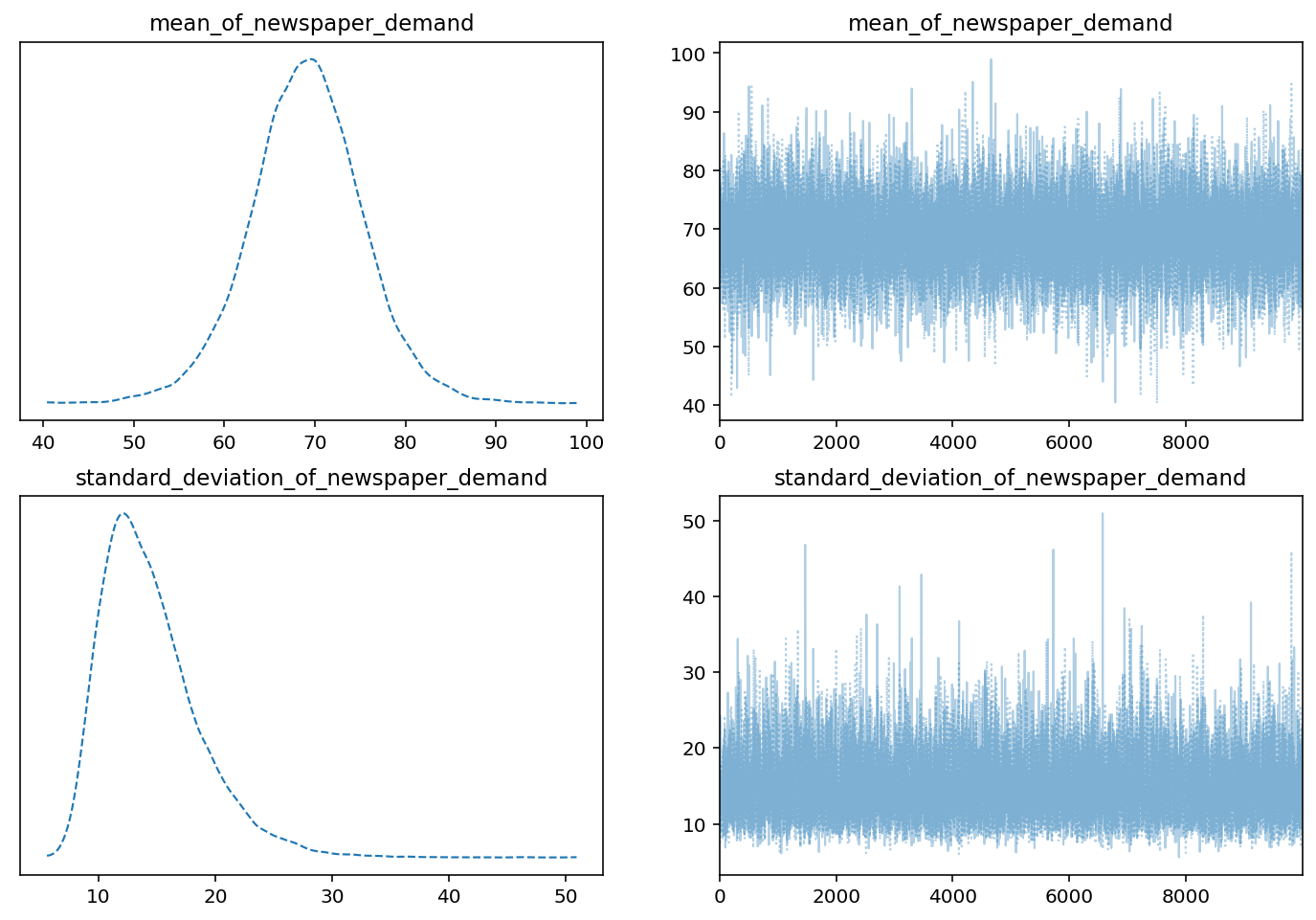And if we were doing more inference:

az.summary: Output High level summary statistics

In :
az.summary(idata, round_to=2)

Out:
mean sd hdi_3% hdi_97% mcse_mean mcse_sd ess_bulk ess_tail r_hat
mean_of_newspaper_demand 69.06 6.26 57.03 80.83 0.06 0.04 10463.73 9522.14 1.0
standard_deviation_of_newspaper_demand 14.51 4.24 7.92 22.46 0.04 0.03 11315.16 11185.45 1.0

### Sample posterior predictive¶

Next we can do a posterior predictive sample with pm.sample_posterior_predictive() and add the extend_inferencedata flag to add the posterior_predictive data as a new category with in our original arviz.InferenceData object.

In :
with newsvendor:
#     posterior_predictive = pm.sample_posterior_predictive(idata, progressbar=True)
pm.sample_posterior_predictive(idata, extend_inferencedata=True)

100.00% [20000/20000 00:03<00:00]
In :
idata.posterior_predictive

Out:
<xarray.Dataset>
Dimensions:       (chain: 2, draw: 10000, demand_dim_0: 5)
Coordinates:
* chain         (chain) int64 0 1
* draw          (draw) int64 0 1 2 3 4 5 6 ... 9994 9995 9996 9997 9998 9999
* demand_dim_0  (demand_dim_0) int64 0 1 2 3 4
Data variables:
demand        (chain, draw, demand_dim_0) float64 30.64 72.65 ... 69.83
Attributes:
created_at:                 2022-06-12T23:11:32.308825
arviz_version:              0.12.1
inference_library:          pymc
inference_library_version:  4.0.0

az.plot_posterior: Pretty-plot the posterior using the style of Kruschke

In :
inf_data = az.convert_to_dataset(idata)
az.plot_posterior(inf_data)

Out:
array([<AxesSubplot:title={'center':'mean_of_newspaper_demand'}>,
<AxesSubplot:title={'center':'standard_deviation_of_newspaper_demand'}>],
dtype=object)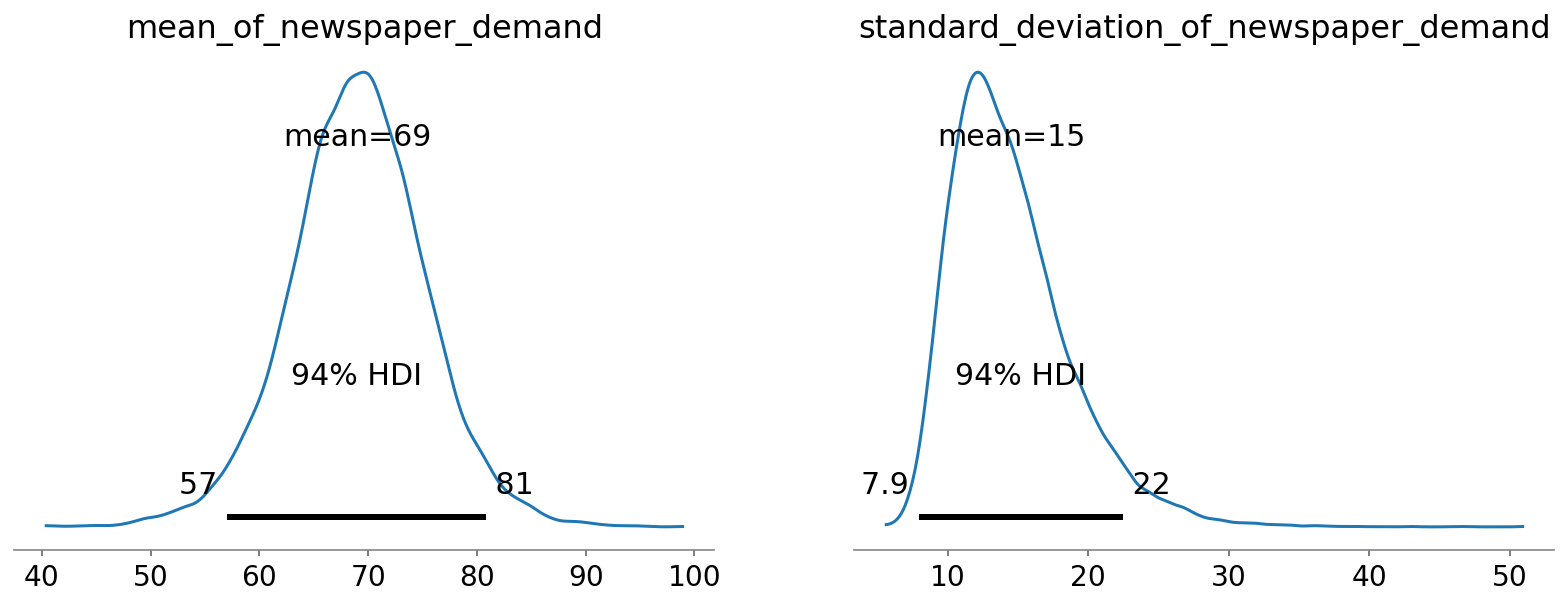Ravin's next point here is that we're using Bayesian Modeling to get all possible values of the demand we could see (given the 5 days of demand we've seen to date) and assign probabilities to each demand scenario.

He illustrates this below by showing a sample of 3 possible demand distributions:

In :
# Get random samples of possible distributions of demand and plot them. Used seed values 0, 10, 15

fig, ax = plt.subplots(figsize=(10, 6))

for seed in [0, 10, 23]:
np.random.seed(seed)
mean = np.random.choice(idata.posterior["mean_of_newspaper_demand"].values.flatten())
std = np.random.choice(idata.posterior["standard_deviation_of_newspaper_demand"].values.flatten())

x = np.linspace(20, 120, 100)
y = stats.norm(mean, std).pdf(x)

ax.plot(x,y)
ax.set_yticks([]);

ax.set_title("Estimated DISTRIBUTIONS of Newspaper Demand \n estimates of parameters");
ax.set_xlabel("Possible demand values of newspapers");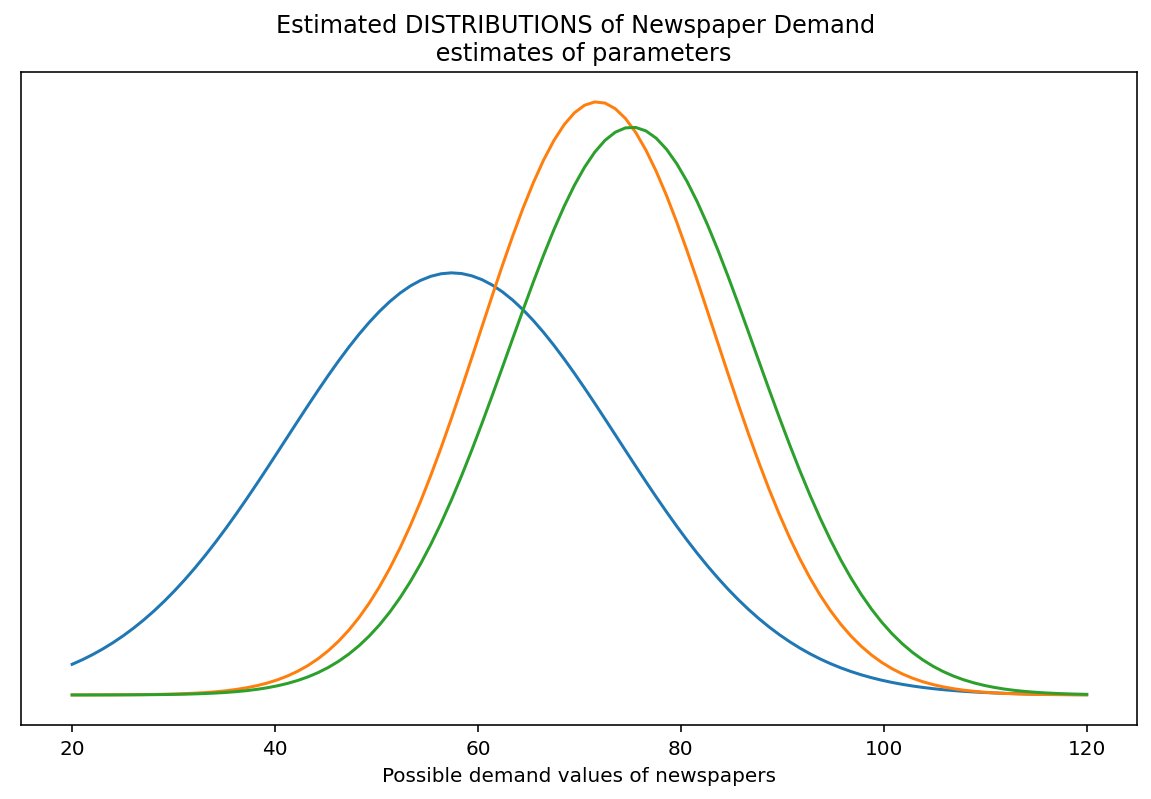Now, we can plot the posterior distribution of possible daily demand:

In :
fig, ax = plt.subplots(figsize=(14, 6))
bayesian_demand_estimates = idata.posterior_predictive["demand"].values.flatten()

az.plot_dist(bayesian_demand_estimates, kind="hist", ax=ax)
ax.set_title("Posterior Predictive Distribution of possible Daily Demands")
ax.set_yticks([]);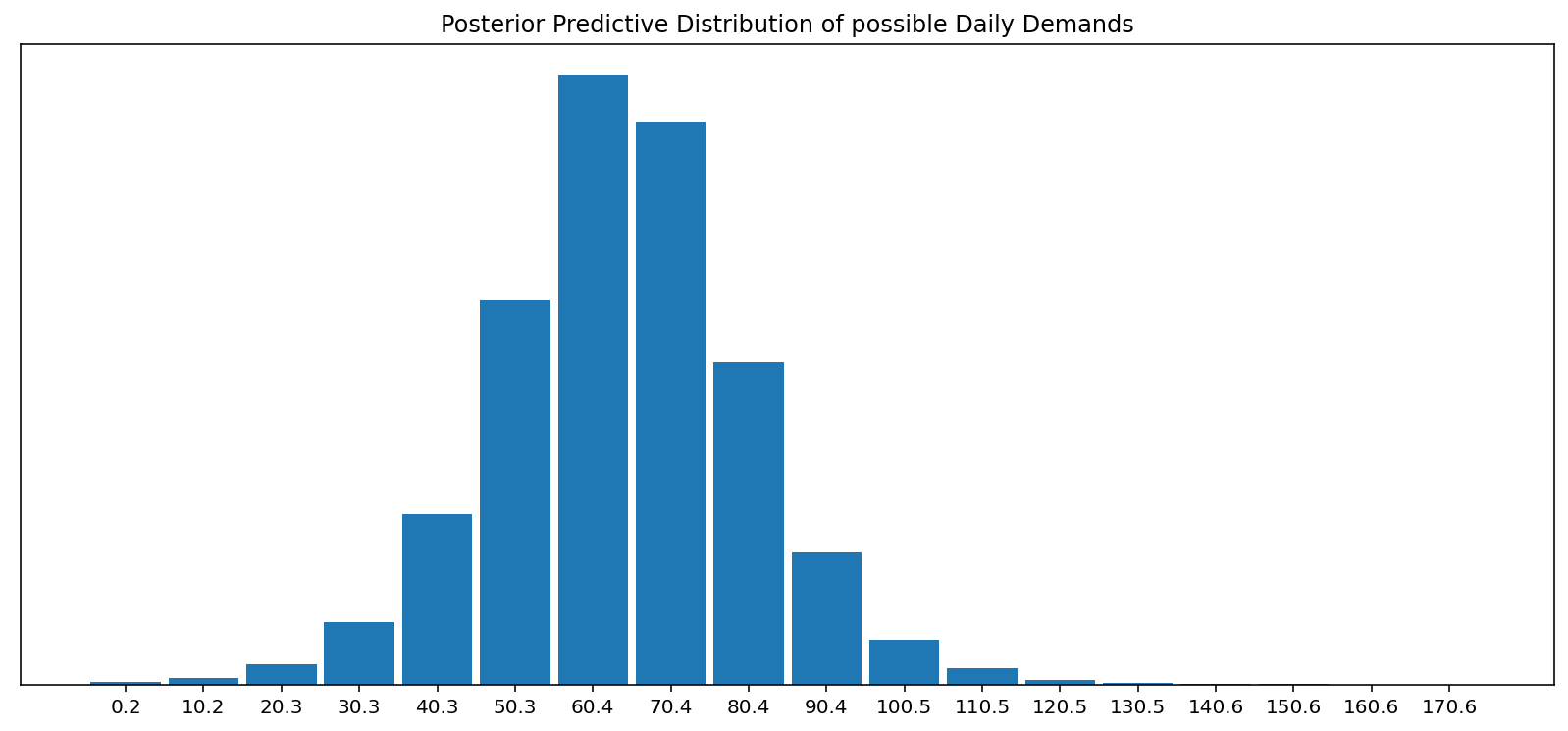## Optimize over all possible demand scenarios¶

Now we can optimize over ALL of the possible demand scenarios

In :
bayesian_demand_estimates = idata.posterior_predictive["demand"].values.flatten()
opt_stoch = optimize.minimize_scalar(objective, bounds=(0, np.inf), args=(bayesian_demand_estimates,))

In :
bayes_opt = opt_stoch.x
f"Optimal inventory from Bayesian demand estimation and optimizer is {np.round(bayes_opt)} newspapers"

Out:
'Optimal inventory from Bayesian demand estimation and optimizer is 61.0 newspapers'

After calculating the optimal inventory strategy, we can then test this strategy on the 10 days of unseen demand and calculate the profit:

In :
objective_func_value = objective(opt_stoch.x, demand_unseen)
f"Testing inventory choice over unseen days yields {objective_func_value} units. Lower is better"

Out:
'Testing inventory choice over unseen days yields -122.45445422518031 units. Lower is better'